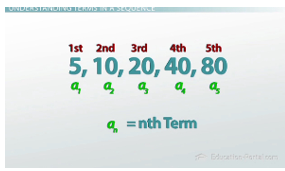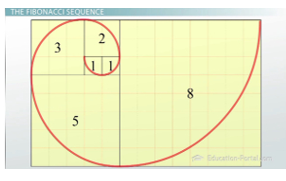# What is a Mathematical Sequence?

#### Video Lesson on What is a Mathematical Sequence?

This lesson video Not available at this time available video coming soon

### What is a Mathematical Sequence?

Math is often the most fun when it acts like a puzzle to be solved. The branch of math where this is the most true involves sequences. Get an introduction to the basics and important vocabulary, as well as learn where sequences appear in nature!

### What Are Sequences?

Sitting in my first college math class at UC Santa Cruz, I was asked by the professor, what is math? I had never really thought about that before and didn't have an answer, but eventually the class came up with a definition that I really liked and have never forgot: math is the study of patterns. While this is true about all areas of math, the branch of math where this is the most obvious is called sequences.

A sequence is just a set of things (usually numbers) that make a pattern. We could have a simple sequence like 1, 2, 3, 4, 5… or a more complicated one like 5, 10, 20, 40, 80… But no matter whether the sequence is simple or complicated, having an agreed upon set of vocabulary is going to be really important.

Looking at our first two examples, you'll notice that I put a '…' at the end of the sequence. This implies that each sequence is an infinite sequence and never stops. But we can also have finite sequences that only have a set number of entries; -10, -5, 0, 5 for example. The sequence stops after we come to the fourth entry.

### Understanding Terms in a SequenceThe letter a followed by a subscript represents each term in the sequence

Another one of the most basic things that should be understood about sequences is that they are made up of terms. Again, going back to one of our first examples, 5, 10, 20, 40, 80…, the pattern begins with the number 5, which means that the first term is 5. That makes the second term 10, the third term 20, the fourth term 40, and so on. Notice that I am using the letter a and then a 'subscript' to describe each term. That mean that a subscript 2 stands for the 2nd term, and a3 stands for the 3rd term and a4, the fourth term, and so on. This means that a25 would be the 25th term and we can even say a subscript n is the 'nth' term, and we're going to use this to mean any generic term. So it'll give me a rule that I can substitute a number in that will tell me any term that I want. We'll get into how to come up with these generic rules for the 'nth' term in later lessons.

### Kinds of Sequences

While this lesson is more about the vocabulary behind sequences, it's also worth mentioning a few of the special ones.

The two most common sequences are called arithmetic and geometric sequences, and in fact all the examples we've looked at so far fall into either one of these two categories. Arithmetic sequences are patterns formed with repeated addition. Our example 1, 2, 3, 4, 5… and on and on is an example of an arithmetic sequence because we were continually adding 1 to get each new term.

Geometric sequences are two sequences that are formed with repeated multiplication. So 5, 10, 20, 40, 80… and on an on, was geometric because we simply multiplied by 2 to find each next term.

### The Fibonacci Sequence

One of the most famous sequences is called the Fibonacci sequence. This sequence is found by adding the previous two entries to come up with the next one. If we define a1, the first term, to be 0 and a2 to be 1, then a3 is just 0+1, which is 1; and then a4 is 1+1, which is 2; then a5 is 1+2, which is 3, and then 5, 8, 13, 21, 34… and this is an infinite sequence.The Fibonacci sequence is often seen in nature

The reason this sequence has become so well known is that it's actually witnessed in nature. For example, making squares with side lengths equal to the terms in the sequence and then drawing a curve through those squares gives us the shape of a very common seashell you might just find on the beach. It's also the case that flowers are most often found with the number of petals that equal one of the entries in the sequence. Even sunflowers, pine cones and pineapples have spiral patterns that match the Fibonacci sequence. It's really everywhere!

It is also an example of a recursive sequence, which loosely means any sequence that depends on the values of the previous terms.

### Lesson Summary

To review: a sequence is simply a pattern of numbers. There can be finite and infinite sequences. Each entry in a sequence is called a term, and is denoted with the letter a and subscript that says which term it is. Arithmetic sequences are formed with repeated addition while geometric sequences are formed with repeated multiplication. Lastly, the Fibonacci sequence is a well known sequence that is an example of a recursive sequence because it relies on the previous entries to determine the next one.

### More Educational and Fun StuffMore In-depth knowledge about what you need. Detailed about test preparation, English Writing, TOEFL, and IELTS.